# K-Prototypes clustering — for when you’re clustering dynamic, real world data

Clustering is one of the most popular types of unsupervised machine learning. Clustering techniques allow us to group data objects into similar classes in such a way that items within a group share similar characteristics, while items in different groups are not similar at all.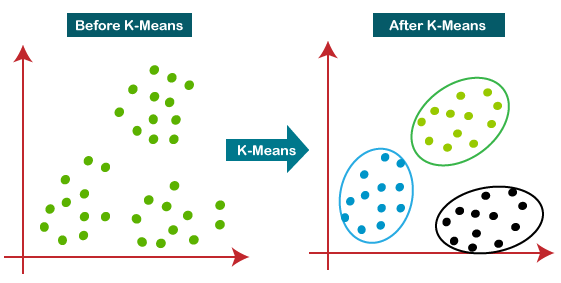cluster analysis 101

It could be said that K-means clustering is the most popular non-hierarchical clustering method available to data scientists today.

For K-means, for each of the predetermined number of K clusters (this is the part that makes it a non-hierarchical algorithm), a seed is selected and each data object (row) in the set is assigned to one of the clusters according to the seed it is closest to. Then for each cluster, a new mean is calculated based on the objects which have been assigned and all objects are once again distributed across all K clusters. The process repeats until there is no change to the computed cluster means, and therefore no objects shift their cluster assignment.

Now, K-means is great for data sets that are all continuous variables, but not so much for when you have data that are categorical. The reason for this is that K-means uses Euclidean distance to measure the similarity of objects… and there aren’t Euclidean distances between data segmented by categories. This can pose a problem, especially when you have a lot of customer data coming from sources outside of something like analytics about their interaction with the company webpage or just looking at past spending habits. Luckily, Zhexue Huang delivered two extensions to the K-means algorithm in 1998, which have allowed for the application of clustering on more real-world data sets that contain a mix of continuous and categorical data.

For the remainder of this post, let’s consider a data set from an old Kaggle competition. The data are attributes for customers of a telecommunication company, and the goal of the competition was to predict whether users would churn (leave the services of the company). I’m using the data here to better understand how the customers grouped, so the goal is less predictive and more understanding the client base.

Here is a partial sample of the data (this is the head of just a few rows):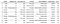partial head of the data frame

The first addition Huang gave us is the K-modes algorithm, which is used to cluster categorical data. Instead of reducing the Euclidean distance between cluster objects and cluster means (centroids) to reduce a cost function, K-modes replaces cluster means with modes and uses a “matching dissimilarity” measure to update cluster modes. This frequency-based method reduces the clustering cost function.

In other words, instead of measuring Euclidean distance on vector data, with the K-modes algorithm the “distance” calculated is simply the number of disagreements between each data point and cluster mode centroid. Looking at the first 10 rows shown above and ignoring the continuous TotalCharges and Churn categories for now, let’s see how that plays out.

The value counts of those rows are as follow: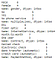value counts

So the mode centroid for the categorical variables (ignoring Churn and Total Charges for now) would be the following:

And then for each object, you could calculate the distance between that centroid and the object by summing the number of dissimilarities there are to the centroid mode.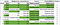Green denotes a match to the ‘Mode’ row

Rows 5 and 8 are the least similar, where their distance of 4 comes from the fact that of the 5 mode characteristics of the group, they only match 1 (it happens to be Month-to-month billing for both). Rows 2 and 10 both have a distance of 0 because they match the group mode identically.

For the whole process, each object has a mode distance measure calculated for each of K centroid modes (we’ve only shown the first centroid above). If the distance between the object and it’s assigned centroid was greater than the distance to another centroid, the object would be reassigned to another centroid. Similar to K-means, this process is repeated until there are no more object re-assignments and group modes are stable. This is the gist of K-modes.

This brings us to K-prototypes, which is simply a joining of the K-means and the K-modes methods.

Finding the optimal K for number of clusters can be tricky, and there are a few methods to do so. One method is by using the Calinski-Harabasz score. The Calinski-Harabesz score is simply a calculation of the variance ratio, which is the ratio of the inter-cluster variance (dispersion between points in a cluster) to the intra-cluster variance (the dispersion between clusters). It is ideal to have a higher score, as that indicates a better performance. Use of CH will be featured in a future post.

Another way to find the optimal number for K is to construct an elbow plot, which will show at which number of K clusters the cost begins decreasing in a linear fashion. For the K-prototypes function, cost is defined as the sum distance of all points to their respective cluster centroids.

In addition to the number of clusters K, we have to determine how to select the starting points for the clusters. There are two methods to initialize the clusters with K-Prototypes, Huang and Cao. Selecting ‘Huang’ as the init, the model will select the first k distinct objects from the data set as initial k-modes and then assign the most frequent categories equally to the initial k-modes. The ‘Cao’ approach selects prototypes for each data object based on the density of the data point and the dissimilarity value.

In the below code, I construct an elbow plot to determine the optimal K by doing the following:

Here is the code and the plot:

`costs = []n_clusters = []cat_cols = [0, 1, 2, 3, 4, 5, 6, 7, 8, 9, 10, 11, 12, 14]for i in tqdm(range(2, 10)):    try:        kproto = KPrototypes(n_clusters=i, init='Huang', verbose=2)        clusters = kproto.fit_predict(data_corr, categorical=cat_cols)        costs.append(kproto.cost_)        n_clusters.append(i)    except:        print(f"Can't cluster with {i} clusters")fig = go.Figure(data=go.Scatter(x=n_clusters, y=costs))fig.show()`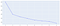Elbow plot

**Side note — you can see that I run my range in tqdm, which provides a cool progress bar while the code runs. Read more about it here!**

Typically I would select 3 clusters, as that is where the cost flattens significantly, however when I ran that, all of the clusters had a mode of ‘No’ for Churn, which is a key feature we are interested in, so I ran it with 4 clusters to show these final clusters, which provide us with Group 1 where mode on Churn is ‘Yes’: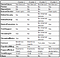Clusters with K-Prototypes (categorical Modes and continuous Means)

Group count is always important in cluster analysis — a group with very few members could be significant and identify outliers, but might not provide information about the whole. The member counts were as follows:

Note also that TotalCharges has been scaled. Because cluster analysis is distance based, it is important to scale all numerical data. Other continuous variables in this data set would also have been scaled had they not been removed due to collinearity. Read another of my posts that discusses more instances of scaling data here.

As you can see, there are some interesting differences. While two groups are more likely to have Fiber Optic than DSL (Groups 1 & 4 vs Group 3), there is a split between Groups 1 and 3 when we look at the services they elect. The customers in Group 1 are churning more frequently than Group 3, however they do not pay for extras like online security, backup, tech support, or device protection.

Senior Citizens do not seem to be the majority in any of the cluster groups, however many of the trends seen in Group 3 align with what we saw for Seniors during data exploration prior to modeling — no internet service, long contracts, no extra frill services, and lowest overall total charges.

There are certainly more insights to gain from this information. We could run predictive models on this data and determine whether this clustering could point to customers who were most likely to leave. We can explore the importance each variable has on determining which cluster the object fall into — I will likely go through those steps in a future post and use the SHAP library to do so.

If you would like to see the full code, including data sourcing, exploration, cleaning, and analysis, check out my Github here. Thank you for reading!